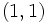# Subgroup structure of direct product of Z4 and Z2

This article gives specific information, namely, subgroup structure, about a particular group, namely: direct product of Z4 and Z2.
View subgroup structure of particular groups | View other specific information about direct product of Z4 and Z2

The direct product of Z4 and Z2 is an abelian group of order eight obtained as the external direct product of cyclic group:Z4 and cyclic group:Z2.

For simplicity, we denote the elements of this group as ordered pairs where the first entry is an integer taken modulo 4 and the second entry is an integer taken modulo 2, with coordinate-wise addition. The group thus has the following eight elements:$\! \{ (0,0), (1,0), (2,0), (3,0), (0,1), (1,1), (2,1), (3,1) \}$

## Tables for quick information

### Table classifying subgroups up to automorphisms

Automorphism class of subgroups List of subgroups Isomorphism class Order of subgroups Index of subgroups Number of conjugacy classes (=1 iff automorph-conjugate subgroup) Size of each conjugacy class (=1 iff normal subgroup) Total number of subgroups (=1 iff characteristic subgroup) Isomorphism class of quotient
trivial subgroup$\{ (0,0) \}$ trivial group 1 8 1 1 1 direct product of Z4 and Z2
first agemo subgroup of direct product of Z4 and Z2$\{ (0,0), (2,0) \}$ cyclic group:Z2 2 4 1 1 1 Klein four-group
non-characteristic order two subgroups of direct product of Z4 and Z2$\{ (0,0), (0,1) \}, \{ (0,0), (2,1) \}$ cyclic group:Z2 2 4 2 1 2 cyclic group:Z4
first omega subgroup of direct product of Z4 and Z2$\{ (0,0), (2,0), (0,1), (2,1) \}$ Klein four-group 4 2 1 1 1 cyclic group:Z2
Z4 in direct product of Z4 and Z2$\{ (0,0), (1,0), (2,0), (3,0) \}$,$(0,0), (1,1), (2,0), (3,1) \}$ cyclic group:Z4 4 2 2 1 2 cyclic group:Z2
whole group all elements direct product of Z4 and Z2 8 1 1 1 1 trivial group

### Table classifying isomorphism types of subgroups

Group name GAP ID Occurrences as subgroup Conjugacy classes of occurrence as subgroup Automorphism classes of occurrence as subgroup Occurrences as normal subgroup Occurrences as characteristic subgroup
Trivial group$(1,1)$ 1 1 1 1 1
Cyclic group:Z2$(2,1)$ 3 3 2 3 1
Cyclic group:Z4$(4,1)$ 2 2 1 2 0
Klein four-group$(4,2)$ 1 1 1 1 1
Direct product of Z4 and Z2$(8,2)$ 1 1 1 1 1
Total -- 8 8 6 8 4

### Table listing number of subgroups by order

Group order Occurrences as subgroup Conjugacy classes of occurrence as subgroup Automorphism classes of occurrence as subgroup Occurrences as normal subgroup Occurrences as characteristic subgroup$1$ 1 1 1 1 1$2$ 3 3 2 3 1$4$ 3 3 2 3 1$8$ 1 1 1 1 1
Total 8 8 6 8 4

## The subgroup comprising the squares (type (2))

### Subgroup-defining functions yielding this subgroup

• Frattini subgroup: The Frattini subgroup of a group of prime power order is the smallest normal subgroup for which the quotient is elementary abelian. For an abelian group of prime power order, the Frattini subgroup is the group comprising the$p^{th}$ powers.
• first agemo subgroup: This is the group generated by the$p^{th}$ powers, in this case the squares. The first agemo subgroup equals the Frattini subgroup for all abelian$p$-groups.